# Journey from Gas Clouds to Stars

• June, 2021

Today we are going to learn about how jeans criterion and magnetohydrodynamics works in astrophysics. but before that first let's what this big words means..

jeans criterion - It simply means Jeans instability and magnetohydrodynamics is the branch of physics that studies the behaviour of an electrically conducting fluid (such as a plasma or molten metal) acted on by a magnetic field.

Now this is very large topic so we can't cover everything in the blog but we are going to flash light upon how this works in astrophysics. best example of this topic in universe is Formation Of Stars from clouds. So we are going to learn in the blog.

#### How Gas Clouds become Star

We believe that stars form when a portion of a molecular cloud collapses gravitationally. Since we have seen that this collapse is resisted by various things, and since we see evidence for many molecular clouds that have not collapsed, it is clear that the collapse initiating star formation occurs only under some circumstances. Can we find a simple condition that tells us when a cloud becomes unstable to collapse?

This question was answered in a very simple model by James Jeans , who showed that for a cloud of a given radius and temperature, there is a critical mass that is now called the Jeans mass If it is exceeded, the cloud becomes unstable to collapse. The Jeans mass depends on the radius of the cloud, its temperature, and the average mass of the particles in the cloud.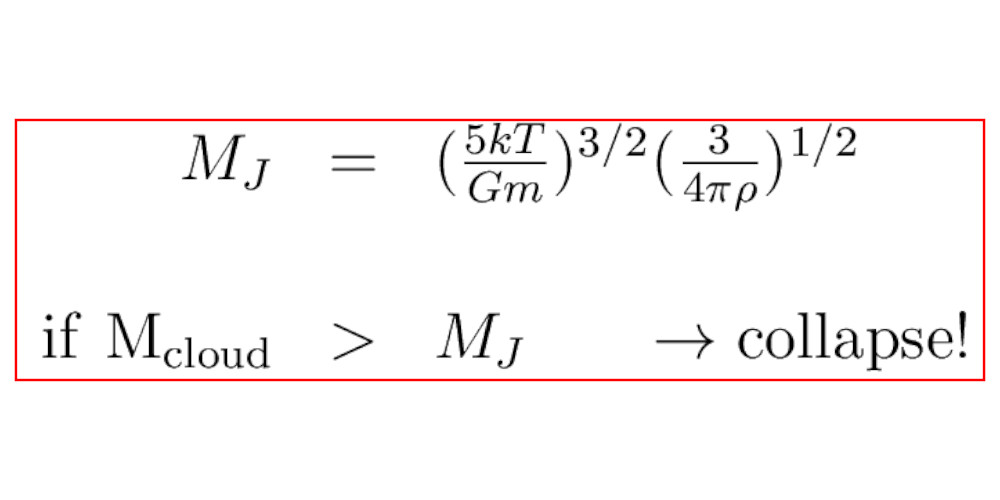Today we are going to learn how James jeans comes with the equation. To understand few of the steps you might require basic knowledge of mathematics and calculus. Even if you don't have that don't worry i try to explain it as simple as possible.

Now suppose you have a finite collection of point particles interacting gravitationally via good old Newtonian mechanics. And suppose that:
1. The time averages of the total kinetic energy and the total potential energy are well-defined.
2. The positions and velocities of the particles are bounded for all time.
Then we have

# E = U/2

where E is the time average of the total kinetic energy, and U is the time average of the total potential energy.

This is a special case of something called the "virial theorem",
The virial theorem states that, for a stable, self-gravitating, spherical distribution of equal mass objects (stars, galaxies, etc), the total kinetic energy of the objects is equal to minus 1/2 times the total gravitational potential energy

Now let's find Jeans equation from the equation.
E = U/2
Now we know that total energy is the sum of potential energy (U) plus kinetic energy (K).
K + U = E

Now put the value of E = U/2 into K + U = E we will get ..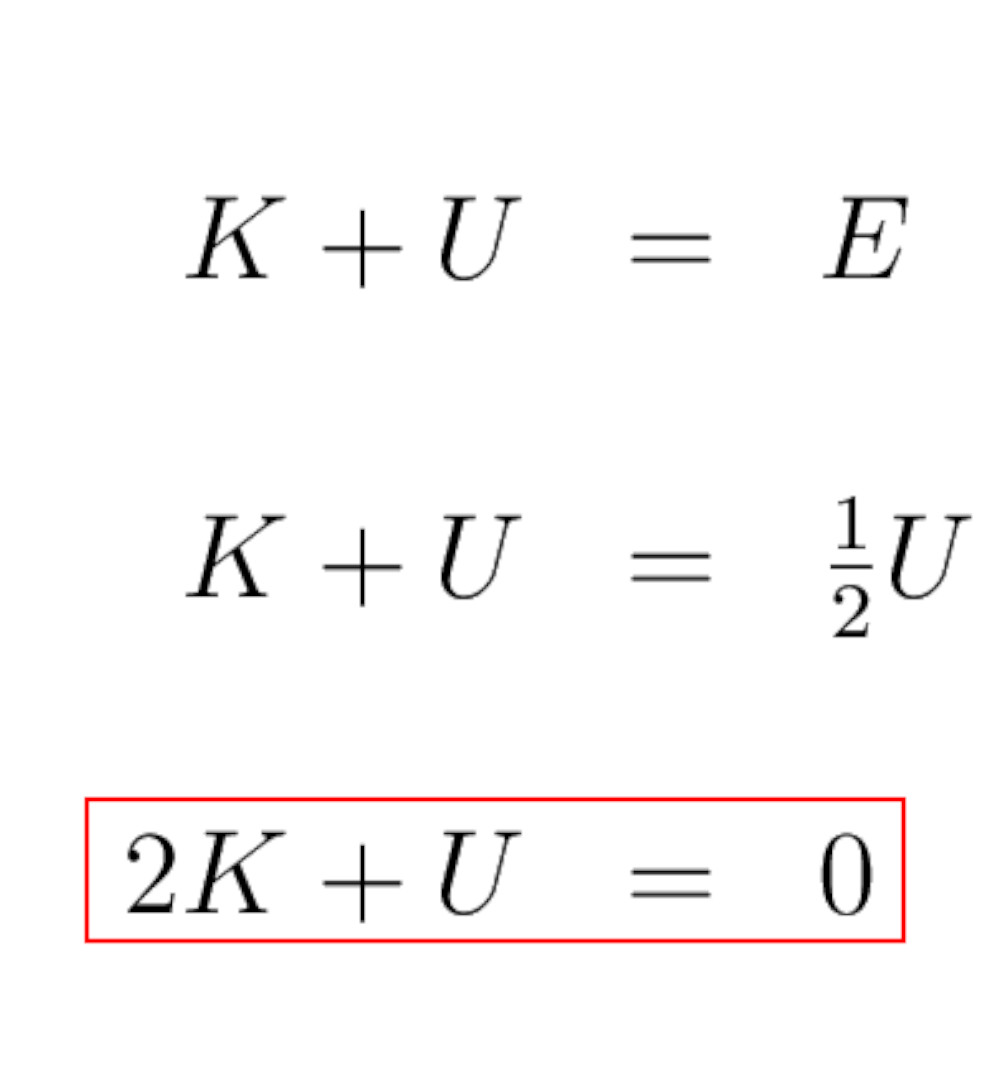What is K, the kinetic energy? In gas clouds, the kinetic energy is due to the motions of the atoms which make up the cloud, so if there are N total atoms in the cloud the kinetic energy is..
The average translational kinetic energy of a molecule is equivalent to 3/2kT and is called thermal energy and for N atoms ...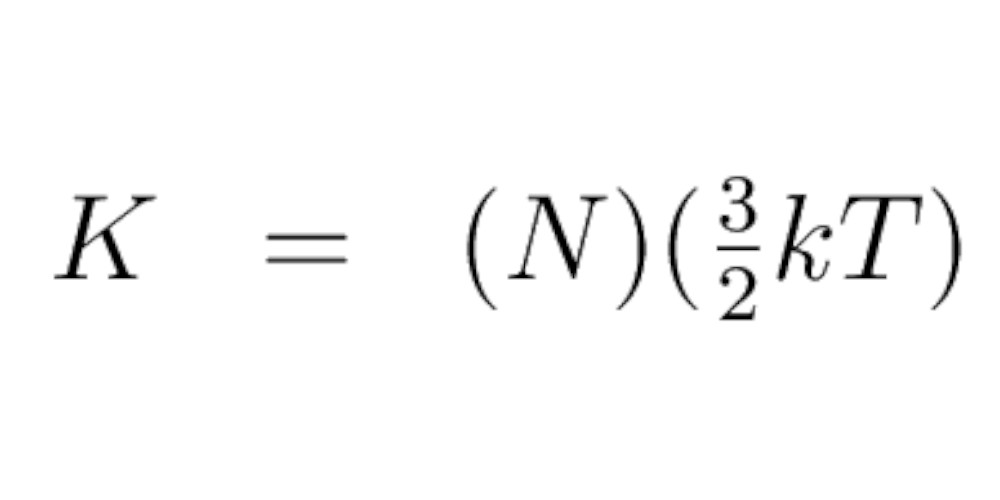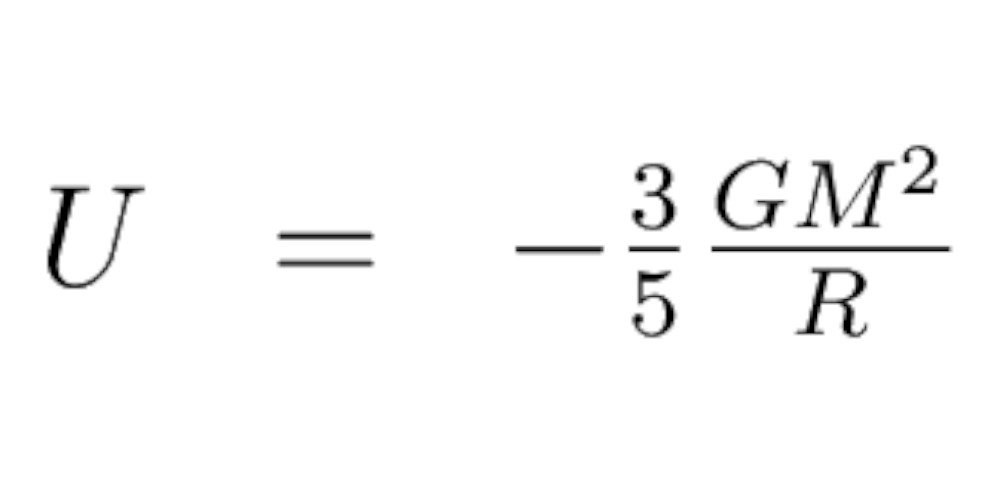And now gravitational potential energy of a uniform sphere means Sun's gravitational potential energy be powering the Sun - is - U
If you are interested in learning about how this equation derives from Sun as a star click here
Now if we put values of U and K in previous equation we will get this new version of viral theoremNow -
- If the equality holds, the system is in virial equilibrium
- If the left side is bigger, kinetic (thermal) energy wins over gravity: expansion
- If the right side is bigger, gravity wins: collapse!
we want to have the cloud collapse, we have to have this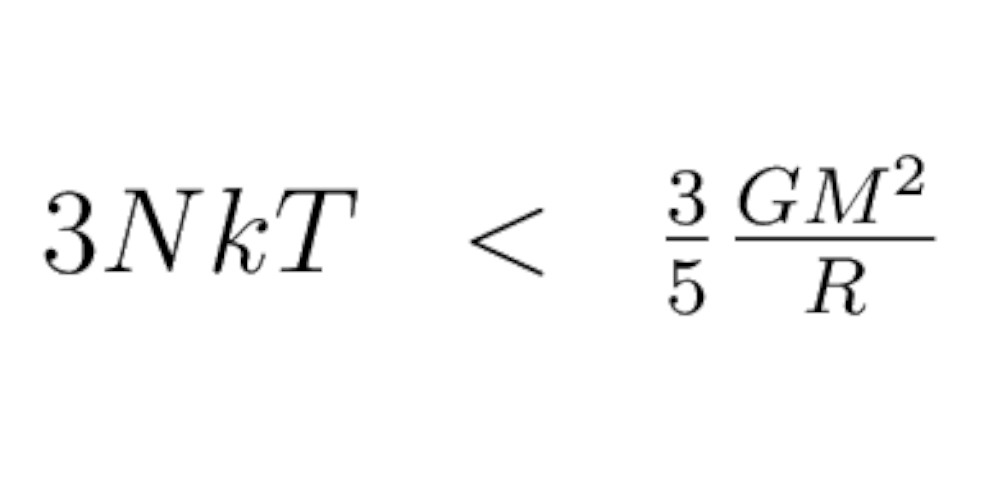So let's substitute. The number of particles is simply the mass of the cloud divided by the mass per particle and

Assuming the cloud has a constant density tho, the size of the cloud can be related to the mass and density by...Plugging these numbers into the collapse criterion equation and doing some math, we get the condition for collapse: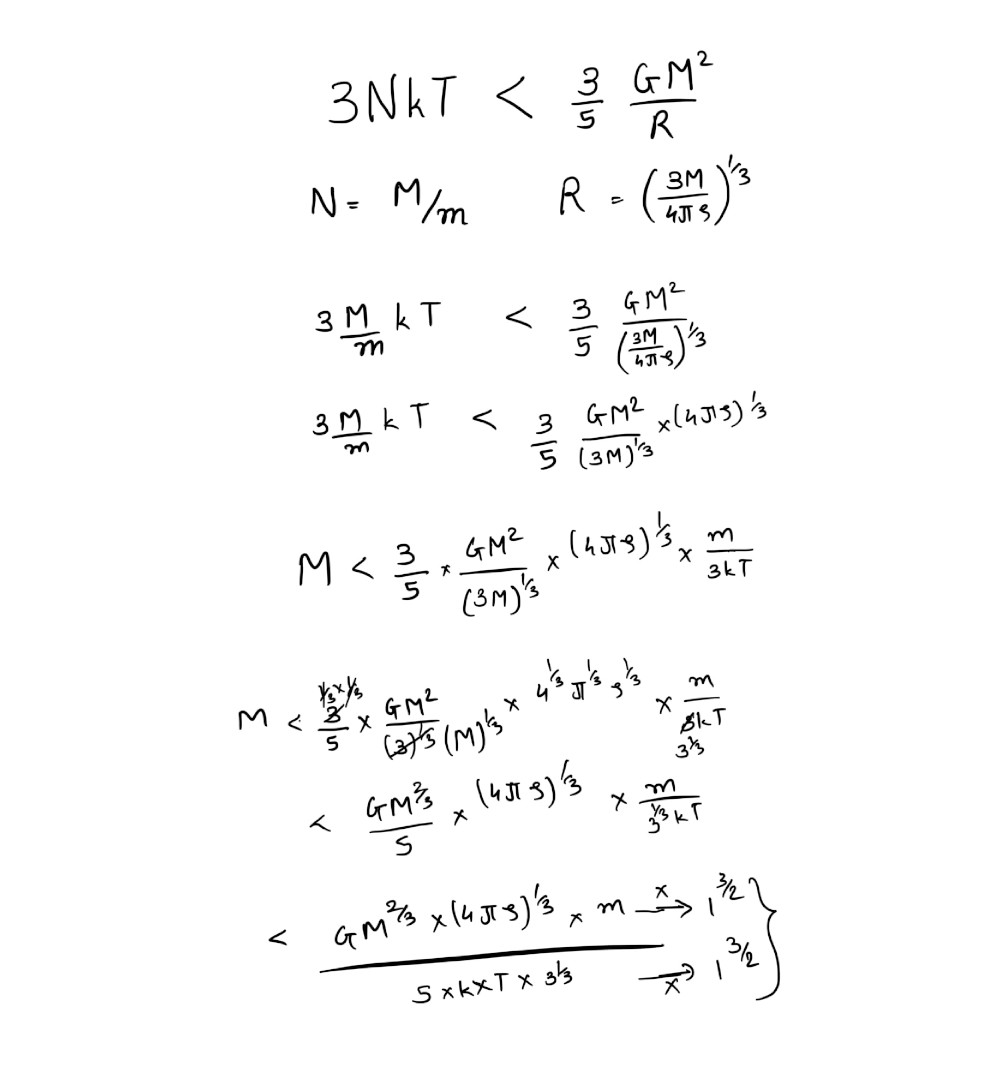Call this number the Jean's mass, then we can say the cloud will collapse if its mass is bigger than the Jean's mass.
This is called the Jean's criterion:Blog Topic suggested By Priyanshu Upadhyay